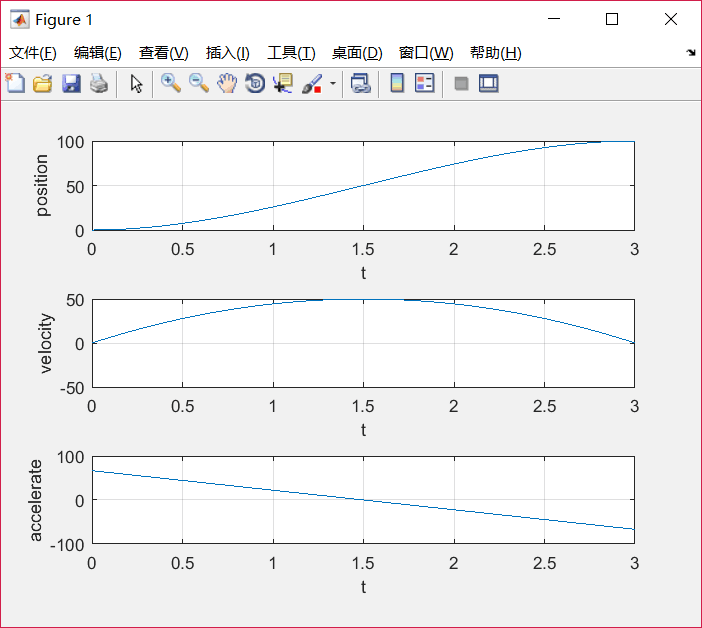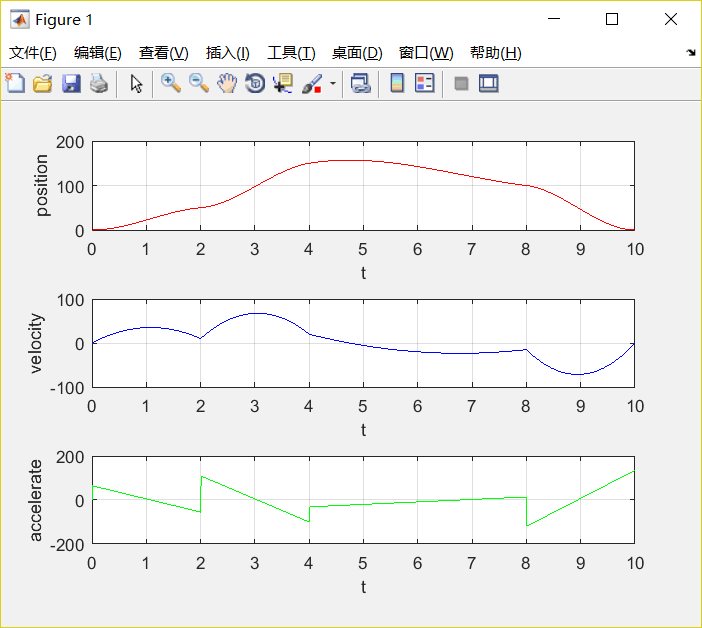•5星
133KB weixin_43328149 2021-06-20 16:55:21
•5星
6KB weixin_43328149 2021-06-04 16:14:02
• 机器人轨迹规划—三次多项式插值轨迹 轨迹规划 三次多项式插值 python 机器人学

三次多项式插值轨迹 梯形速度曲线轨迹 双S形速度曲线轨迹 多个自由度轨迹的时间同步 在线轨迹规划 多项式在线轨迹规划 梯形在线轨迹规划 双S形在线轨迹规划 非线性实时轨迹滤波 多点轨迹（Multi-point) 三次...

机器人轨迹

这一系列轨迹教程将主要包括以下内容：

• 点到点轨迹（P2P）

• 在线轨迹规划

• 多项式在线轨迹规划
• 梯形在线轨迹规划
• 双S形在线轨迹规划
• 非线性实时轨迹滤波
• 多点轨迹（Multi-point)

• 三次样条曲线（cubic spline）
• 贝赛尔曲线（Bezier Curve）
• B样条曲线（BSpline）
• 时间最优轨迹

• 三次样条时间最优轨迹
• 任意路径下的时间最优轨迹
• 时间最优停止轨迹
• 不约束路径
• 有路径约束

展开全文xiaozisheng2008_ 2021-02-28 18:45:37
• 机器人学之运动学笔记【5】—— 机械臂轨迹规划【1】 轨迹：机械臂的末端点或操作点的位置、速度、加速度对时间的历程

轨迹：机械臂的末端点或操作点的位置、速度、加速度对时间的历程
理想轨迹：smooth path，位置以及速度甚至加速度都连续的轨迹1. Joint-space 下的轨迹规划用图形描述上述过程：总结起来就是：

由笛卡尔空间变换到关节空间，中间需要逆运动学求解，找出目标姿态对应的各个关节角度，然后轨迹规划，描绘出各个关节角度随时间的变动情况，最后用正向运动学求解一下，看看所求轨迹是否和理论轨迹相符，再检验一下轨迹的可行性。

2. Cartesian-space下的轨迹规划

第一步和 Joint space 时一样，因为第一步其实是和轨迹规划的部分是独立的，是对需求的描述。3. 轨迹规划方法一：Cubic Polynomials(三次多项式)• 每两个点之间用不同参数的函数来规划，找到函数把两个点串起来
• 任何两端相接的轨迹都要满足 smooth 的条件，也就是说每段函数头尾处的条件要定义清楚，所以一段函数、两个边界、四个条件。因此需要三次多项式来规划轨迹（因为三次多项式有4个参数可供我们选择）

3.1 解单段 Cubic polynomial对于一条三次多项式曲线，包含a0、a1、a2、a3四个未知参数，使用 t‘ = t - t(i) 处理方法可以简约计算，结合四个边界条件，可以很快求得四个未知参数的值。3.2 解多段 Cubic Polynomials

如果对于中间的一个 via point ，位置我们可能会已知，那么速度边界条件该如何定义呢？或者说如何选择速度条件呢？以这两个方式，不同区段的 Cubic polynomials 可以分开求解。求得两条三次多项式曲线，包含8个未知数，通过下图所示：四个位置边界条件、两个速度边界条件、一个速度连续条件、一个加速度连续条件总共8个条件，就可以求得这8个未知参数，从而确定两条三次多项式曲线。3.3 推广到一般情况 General cubic spline function这里设置成N+1是为了造成N条线段，每条线段有四个未知参数，所以总共有4N个参数需要求解，为此还需要找到4N个方程联立求解。3.4 练习3.4.1 以cubic polynomials 在 Cartesian-space 下规划轨迹每个自由度包含三条三次多项式曲线，而每条曲线对应4个未知数，所以一个自由度要确定12个未知参数。
用矩阵解法，前六个是位置条件，第七第八个条件是一开始的速度和最后的速度，第九第十个条件是第一个 via point 的速度和加速度条件，倒数后两个是第二个 via point 的速度加速度条件。3.4.2 以cubic polynomials 在 Joint-space 下规划轨迹4. 位置、速度、加速度都需要规划的情况展开全文huangjunsheng123 2020-10-31 19:46:06
• 机器人轨迹规划——多项式轨迹实战 轨迹规划 多项式轨迹 c++ pybind

三次多项式插值轨迹 五次多项式插值轨迹 多项式轨迹实战 梯形速度曲线轨迹 双S形速度曲线轨迹 多个自由度轨迹的时间同步 在线轨迹规划 多项式在线轨迹规划 梯形在线轨迹规划 双S形在线轨迹规划 非线性实时轨迹...

机器人轨迹

这一系列轨迹教程将主要包括以下内容：

• 点到点轨迹（P2P）

• 在线轨迹规划

• 多项式在线轨迹规划
• 梯形在线轨迹规划
• 双S形在线轨迹规划
• 非线性实时轨迹滤波
• 多点轨迹（Multi-point)

• 三次样条曲线（cubic spline）
• 贝赛尔曲线（Bezier Curve）
• B样条曲线（BSpline）
• 时间最优轨迹

• 三次样条时间最优轨迹
• 任意路径下的时间最优轨迹
• 时间最优停止轨迹
• 不约束路径
• 有路径约束

[TOC]

展开全文xiaozisheng2008_ 2021-03-27 11:50:27
• 分段三次样条：通过拼接两个三次多项式进行机器人运动的轨迹规划-matlab开发 matlab

2KB weixin_38529397 2021-06-01 10:22:21
• 六轴机器人轨迹规划之三次多项式轨迹插值 插值算法 三次多项式插值

1.轨迹规划的定义 轨迹规划(trajectory planning)是运动规划(motion planning)研究的主要内容。运动规划指的是运动插补，在起始点和终止点之间插入中间点序列，实现沿着轨迹的平稳运动。运动控制包含路径规划(path ...

1.轨迹规划的定义
轨迹规划(trajectory planning)是运动规划(motion planning)研究的主要内容。运动规划指的是运动插补，在起始点和终止点之间插入中间点序列，实现沿着轨迹的平稳运动。运动控制包含路径规划(path planning)和轨迹规划，路径规划是规划位置，在起终点之间经过的路径点，轨迹规划是规划时间，将路径点与时间相对应。
对于我们的六轴机器人而言轨迹规划可以分为：关节空间轨迹规划和笛卡尔空间轨迹规划。关节空间轨迹规划是把机器人的关节变量变换成跟时间的函数，然后对角速度和角加速度进行约束。笛卡尔空间轨迹规划是把机器人末端在笛卡尔空间的位移、速度和加速度变换成跟时间的函数关系。
2.数学基础
三次多项式插值（适用于起点和终点速度为零的情况，约束关节在起点和终点的角度值，规定轨迹两端点位置角速度为定值）。
设关节角满足下式

θ(t)=a0+a1t+a2t2+a3t3θ˙(t)=a1+2a2t+3a3t2θ¨(t)=2a2+6a3t { θ ( t ) = a 0 + a 1 t + a 2 t 2 + a 3 t 3 θ ˙ ( t ) = a 1 + 2 a 2 t + 3 a 3 t 2 θ ¨ ( t ) = 2 a 2 + 6 a 3 t

将相邻两个点看作一小段轨迹的起点和终点分别用 θ0 θ 0 $\theta_0$ θf θ f $\theta_f$表示，约束起始速度为 v0 v 0 $v_0$，终止速度为 vf v f $v_f$
θ(t0)=θ0θ(tf)=θfθ˙(t0)=v0θ˙(tf)=vf { θ ( t 0 ) = θ 0 θ ( t f ) = θ f θ ˙ ( t 0 ) = v 0 θ ˙ ( t f ) = v f

将约束条件代入函数，可以求得系数（为简便计算，设 t0=0 t 0 = 0 $t_0=0$
a0=θ0a1=v0a2=3t2f(θfθ0)1tf(2v0+vf)a3=2t3f(θ0θf)+1t2f(v0+vf) { a 0 = θ 0 a 1 = v 0 a 2 = 3 t f 2 ( θ f − θ 0 ) − 1 t f ( 2 v 0 + v f ) a 3 = 2 t f 3 ( θ 0 − θ f ) + 1 t f 2 ( v 0 + v f )

三次多项式规划轨迹如下
θ(t)=θ0+v0t+[3t2f(θfθ0)1tf(2v0+vf)]t2+[2t3f(θ0θf)+1t2f(v0+vf)]t3θ˙(t)=v0+2[3t2f(θfθ0)1tf(2v0+vf)]t+3[2t3f(θ0θf)+1t2f(v0+vf)]t2θ¨(t)=2[3t2f(θfθ0)1tf(2v0+vf)]+6[2t3f(θ0θf)+1t2f(v0+vf)]t { θ ( t ) = θ 0 + v 0 t + [ 3 t f 2 ( θ f − θ 0 ) − 1 t f ( 2 v 0 + v f ) ] t 2 + [ 2 t f 3 ( θ 0 − θ f ) + 1 t f 2 ( v 0 + v f ) ] t 3 θ ˙ ( t ) = v 0 + 2 [ 3 t f 2 ( θ f − θ 0 ) − 1 t f ( 2 v 0 + v f ) ] t + 3 [ 2 t f 3 ( θ 0 − θ f ) + 1 t f 2 ( v 0 + v f ) ] t 2 θ ¨ ( t ) = 2 [ 3 t f 2 ( θ f − θ 0 ) − 1 t f ( 2 v 0 + v f ) ] + 6 [ 2 t f 3 ( θ 0 − θ f ) + 1 t f 2 ( v 0 + v f ) ] t

当速度为零时适用于一段轨迹的起终点，不为零时适用于一段轨迹的经过点。
3.matlab代码实现

序号位置速度时间
1000
210003
clear;
clc;
q0=0;
q1=100; %指定起止位置
t0=0;
t1=3;%指定起止时间
v0=0;
v1=0;%指定起止速度
a0=q0;
a1=v0;
a2=(3/(t1)^2)*(q1-q0)-(1/t1)*(2*v0+v1);
a3=(2/(t1)^3)*(q0-q1)+(1/t1^2)*(v0+v1);%计算三次多项式系数
t=t0:0.01:t1;
q=a0+a1*t+a2*t.^2+a3*t.^3;%三次多项式插值的位置
v=a1+2*a2*t+3*a3*t.^2;%三次多项式插值的速度
a=2*a2+6*a3*t;%三次多项式插值的加速度
subplot(3,1,1),plot(t,q),xlabel('t'),ylabel('position');grid on;
subplot(3,1,2),plot(t,v),xlabel('t'),ylabel('velocity');grid on;
subplot(3,1,3),plot(t,a),xlabel('t'),ylabel('accelerate');grid on;

下图为插值结果4.含经过点的插值

序号位置速度时间
1000
250103
3150206
4100-1512
50014
clear;
clc;
q_array=[0,50,150,100,0];%指定起止位置
t_array=[0,2,4,8,10];%指定起止时间
v_array=[0,10,20,-15,0];%指定起止速度
t=[t_array(1)];q=[q_array(1)];v=[v_array(1)];a=;%初始状态
for i=1:1:length(q_array)-1;%每一段规划的时间
a0=q_array(i);
a1=v_array(i);
a2=(3/(t_array(i+1)-t_array(i))^2)*(q_array(i+1)-q_array(i))-(1/(t_array(i+1)-t_array(i)))*(2*v_array(i)+v_array(i+1));
a3=(2/(t_array(i+1)-t_array(i))^3)*(q_array(i)-q_array(i+1))+(1/(t_array(i+1)-t_array(i))^2)*(v_array(i)+v_array(i+1));%计算三次多项式系数
ti=t_array(i)+0.001:0.001:t_array(i+1);
qi=a0+a1*(ti-t_array(i))+a2*(ti-t_array(i)).^2+a3*(ti-t_array(i)).^3;
vi=a1+2*a2*(ti-t_array(i))+3*a3*(ti-t_array(i)).^2;
ai=2*a2+6*a3*(ti-t_array(i));
t=[t,ti];q=[q,qi];v=[v,vi];a=[a,ai];
end
subplot(3,1,1),plot(t,q,'r'),xlabel('t'),ylabel('position');grid on;
subplot(3,1,2),plot(t,v,'b'),xlabel('t'),ylabel('velocity');grid on;
subplot(3,1,3),plot(t,a,'g'),xlabel('t'),ylabel('accelerate');grid on;PS:这种三次多项式插值，只要给定离散点位置、速度和时间，就能插补出一段连续平滑的曲线。但是角加速度并不连续，下次为大家介绍高阶多项式插值则可以解决这个问题。

展开全文jldemanman 2018-02-09 14:55:09
• 机器人轨迹规划—五次多项式插值轨迹 机器人学 机器人轨迹 轨迹规划

xiaozisheng2008_ 2021-02-27 11:50:30
• 轨迹规划 - 三次多项式and五次多项式 轨迹规划 三次多项式 五次多项式

ymj7150697 2020-04-23 18:06:49
• 机器人学回炉重造（5-1）：关节空间规划方法——多项式轨迹（三次多项式、五次多项式、抛物线轨迹） 机器人学 关节空间规划 三次多项式 五次多项式

qq_26565435 2019-07-03 11:05:56
• 机械臂建模实战-七次多项式轨迹规划 matlab

weixin_46169171 2021-11-08 22:35:32
• 六轴机器人轨迹规划之五次多项式插值 六轴机器人 轨迹规划 五次多项式插值

jldemanman 2018-02-10 08:51:50
•5星
16.57MB qq_34535738 2018-11-21 10:54:04
• 多项式轨迹--五次多项式轨迹 多项式轨迹 五次多项式

Galaxy_Robot 2020-07-28 22:37:38
• 机器人轨迹规划matlab程序 轨迹规划

2KB fanliuqiang 2017-02-18 15:06:08
• 2KB sunlinsunshine 2018-11-09 10:56:44
• qq_41658212 2020-02-14 10:57:09
• 时间最优轨迹规划（3-5-3次多项式） 算法 粒子群算法 matlab

qq_46107700 2021-10-15 17:30:27
• 机器人控制与轨迹规划 轨迹规划 matlab

qq_37469992 2021-01-03 09:44:12
• weixin_41045354 2021-04-05 18:28:44
• m0_37764065 2020-03-09 10:38:40
• 【机器人技术基础】MATLAB Robotics Toolbox PUMA560 7次多项式取-放轨迹的规划 matlab仿真 PUMA560 轨迹规划

qq_44324181 2021-01-17 20:27:35
• 机器人轨迹规划：三次样条曲线 轨迹规划 三次样条曲线

qq_26565435 2020-05-05 22:47:25
• weixin_29722785 2021-04-29 01:36:46
•5星
133KB weixin_43328149 2021-06-20 16:55:21...